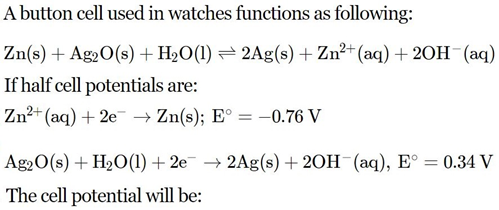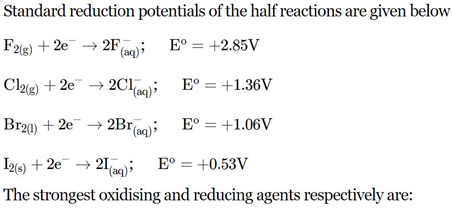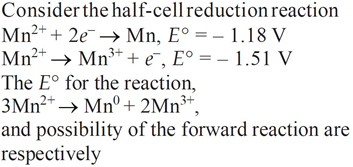# Electrochemistry NEET Previous Year Questions

1. The standard electrode potential (E°) values of Al3+/Al, Ag+/Ag, K+/K and Cr3+/Cr are –1.66 V, 0.80 V, –2.93 V and –0.74 V, respectively. The correct decreasing order of reducing power of the metal is                    (NEET 2019)

(a) Ag > Cr > Al > K             (b) K > Al > Cr > Ag

(c) K > Al > Ag > Cr             (d) Al > K > Ag > Cr

2.(NEET 2013)

(a) 0.84 V                                  (b) 1.34 V

(c) 1.10 V                                  (d) 0.42 V

03.(Mains 2012)

(a) F2 and I                    (b) Br2 and Cl

(c) Cl2 and Br                 (d) Cl2 and I2

4. Standard electrode potentials of three metals X, Y and Z are –1.2 V, + 0.5 V and – 3.0 V respectively. The reducing power of these metals will be     (2011)

(a) Y > Z > X

(b) Y > X > Z

(c) Z > X > Y

(d) X > Y > Z

5. Standard electrode potential for Sn4+/Sn2+couple is +0.15 V and that for the Cr3+ /Couple is –0.74 V. These two couples in their standard state are connected to make a cell. The cell potential will be                                          (2011)

(a) + 1.19 V            (b) + 0.89 V

(c) + 0.18 V            (d) + 1.83 V

6. A solution contains Fe2+, Fe3+ and I ions. This solution was treated with iodine at 35°C. E° for Fe3+ /Fe2+ is + 0.77 V and E° for I2/2I = 0.536 V.  (2011)

The favourable redox reaction is

(a) I2 will be reduced to I

(b) there will be no redox reaction

(c) I will be oxidised to I2

(d) Fe2+ will be oxidised to Fe3+.

7. Consider the following relations for emf of an electrochemical cell            (2011)

(i) EMF of cell = (Oxidation potential of anode) –

(Reduction potential of cathode)

(ii) EMF of cell = (Oxidation potential of anode) + (Reduction potential of cathode)

(iii) EMF of cell = (Reductional potential of anode) + (Reduction potential of cathode)

(iv) EMF of cell = (Oxidation potential of anode) – (Oxidation potential of cathode)

Which of the above relations are correct?

(a) (iii) and (i)               (b) (i) and (ii)

(c) (iii) and (iv)             (d) (ii) and (iv)

8. On the basis of the following E° values, the strongest oxidizing agent is

[Fe(CN)6]4– → [Fe(CN)6]3– + e ;

E° = –0.35 V Fe2+ → Fe3+ + e ;

E° = –0.77 V

(a) Fe3+                            (b) [Fe(CN)6]3–

(c) [Fe(CN)6]4–                (d) Fe2+

10.Fe2+/Fe = – 0.441 V and E°Fe3+/Fe2+ = 0.771 V, the standard EMF of the reaction Fe + 2Fe3+ → 3Fe2+ will be

(a) 0.111 V                       (b) 0.330 V

(c) 1.653 V                        (d) 1.212 V

17. For a cell involving one electron, E°cell = 0.59 V at 298 K, the equilibrium constant for the cell reaction is                                  (NEET 2019)

(a) 1.0 × 1030                             (b) 1.0 × 102

(c) 1.0 × 105                              (d) 1.0 × 1010

18. In the electrochemical cell : Zn|ZnSO4(0.01 M)||CuSO4(1.0 M)|Cu,

the emf of this Daniell cell is E1. When the concentration of ZnSO4 is changed to 1.0 M and that of CuSO4 changed to 0.01 M, the emf changes to E2. From the followings, which one is the relationship between E1 and E2? (Given, RT/F = 0.059)                                                                    (NEET 2017, 2003)

(a) E1 < E2            (b) E1 > E2

(c) E2 = 0 = E1      (d) E1 = E2

19. If the E°cell for a given reaction has a negative value, which of the following gives the correct relationships for the values of ΔG° and Keq ? (NEET 2017, 2011)

(a) ΔG° > 0; Keq < 1                  (b) ΔG° > 0; Keq > 1

(c) ΔG° < 0; Keq > 1                  (d) ΔG° < 0; Keq < 1

20. The pressure of H2 required to make the potential of H2 electrode zero in pure water at 298 K is                                (NEET 2016)

(a) 10–10 atm             (b) 10–4 atm

(c) 10–14 atm         (d) 10–12 atm.

21. A hydrogen gas electrode is made by dipping platinum wire in a solution of HCl of pH = 10 and by passing hydrogen gas around the platinum wire at one atm pressure. The oxidation potential of electrode would be (NEET 2013)

(a) 0.118 V                          (b) 1.18 V

(c) 0.059 V                           (d) 0.59 V

22.(NEET 2013)

(a) – 4.18 V and yes                  (b) + 0.33 V and yes

(c) + 2.69 V and no                  (d) – 2.69 V and no.

23. The Gibbs energy for the decomposition of Al2O3 at 500°C is as follows:

2/3Al2O3 → 4/3Al + O2;     ΔrG = +966 kJ/mol.

The potential difference needed for electrolytic reduction of Al2O3 at 500°C is at least:                                                                           (Mains 2012)

(a) 4.5 V                (b) 3.0 V

(c) 2.5 V                (d) 5.0 V

24.